#Quality Digest

Featured Product
This Week in Quality Digest Live
Statistics Features
Donald J. Wheeler
What do predictable processes have in common with chaos?
William A. Levinson
People can draw the wrong conclusions due to survivor, survey, and bad news bias.
Donald J. Wheeler
Does your approach do what you need?
Paul Laughlin
Correlation vs. causality
Donald J. Wheeler
In spite of what everyone says to the contrary
Statistics News
New capability delivers deeper productivity insights to help manufacturers meet labor challenges
Day and a half workshop to learn, retain, and transfer GD&T knowledge across an organization
Elsmar Cove is a leading forum for quality and standards compliance
InfinityQS’ quality solutions have helped cold food and beverage manufacturers around the world optimize quality and safety
User friendly graphical user interface makes the R-based statistical engine easily accessible to anyone
Collect measurements, visual defect information, simple Go/No-Go situations from any online device
Good quality is adding an average of 11 percent to organizations’ revenue growth
Ability to subscribe with single-user minimum, floating license, and no long-term commitment
A guide for practitioners and managersBio

Statistics

## What Is the Probability That Your Process Is Predictable?

### How to compute a p-value for your process behavior chart

Published: Wednesday, January 9, 2019 - 13:03

Story update 1/15/2019: Thanks to the sharp eye of Dr. Stan Alekman, who spotted an inconsistent value in figure 2, I discovered an error in the program used to construct the table of critical values for the prediction ratio. I have now corrected that problem and updated the entries in the table in figure 2. If you previously downloaded this column, you might want to download the corrected version below.

Software packages use p-values to report the results of many statistical procedures. As a result some people have come to expect a p-value as the outcome of any statistical analysis. This column will tell you how to compute and use a p-value for a process behavior chart.

### Background

Process behavior charts allow you to listen to the voice of your process. They allow you to characterize the process as being operated predictably or unpredictably. They help you to find assignable causes of exceptional variation and thereby to reduce the process variation and increase productivity. However, in this day of instant pudding, rather than taking the time to listen to the voice of the process, people want everything boiled down to a number they can put in the monthly report. At the risk of contributing to this practice, this column tells you how to compute and use a p-value for your process behavior chart.

Process behavior charts were designed for the sequential analysis of a continuing stream of observational data. Here the data generally represent one condition and the purpose of the chart is to identify unplanned changes in the underlying process. After the limits have been computed for some baseline period, they are extended forward and additional points are added to the chart as they become available. Each time we add another point to the chart we are performing an act of analysis, and each of these sequential analyses asks if the current value is consistent with the baseline period.

Because of this sequential nature, a process behavior chart has no fixed risk of a false alarm and no fixed risk of a missed signal. So how can we talk about a p-value for a process behavior chart? We can do this in the same way that we compute all of the other values associated with a process behavior chart—we use the baseline period. We compute the average, the average range, the limits, the capability indexes, and the performance indexes using that fixed amount of data we define as the baseline period. We shall do the same for the p-value.

### What is the probability that your process is predictable?

A p-value is a test statistic that is expressed as a probability. Under the condition that there is no difference between two quantities, a p-value is the probability of getting a result that is more unlikely than the observed result. So small p-values are associated with unlikely events and large p-values are associated with likely events (under the condition that no difference exists).

Here we shall use a p-value to ask the question: "What is the probability that this process was operated predictably during the baseline period?" Since predictable operation provides a rational basis for using that product which we have measured to characterize the product that was not measured, this question of predictability is very important in practice. A small p-value will be an indication that the process is unlikely to have been operated predictably and our extrapolation to the unmeasured product becomes questionable.

Say we have a baseline that consists of k subgroups of size n, so that the total number of data in the baseline is N = nk. (In the case of an XmR chart we define n = 1.) We define the capability and performance indexes as follows:The quantities in these formulas are defined as follows. The difference between the specification limits, USLLSL, is the specified tolerance. The distance to the nearer specification, DNS, is the distance from the average to the nearer specification limit. Sigma(X) denotes any one of several within-subgroup measures of dispersion, such as the average of the subgroup ranges divided by the appropriate bias correction factor, d2. And s is the global standard deviation statistic computed using all N data in the baseline period.

### The predictability ratio

Using a baseline consisting of N data, define the predictability ratio as the capability ratio, Cp, divided by the performance ratio, Pp.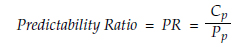Next we compare our observed value for the predictability ratio with the maximum 1-percent critical value for N data (found in figure 2). If your computed predictability ratio exceeds the maximum critical value for N data, you have less than a 1-percent chance that your process has been operated predictably. This is evidence that is strong enough to convince a skeptic that the process was operated unpredictably.

If your predictability ratio is noticeably smaller than the maximum critical value you can say that its p-value will be larger than 1 percent. However, in this case the process may, or may not, have been operated predictably. The only way to judge that a process displays a reasonable degree of predictability is to use the process behavior chart.Figure 1:  The relationship between the predictability ratio and the p-value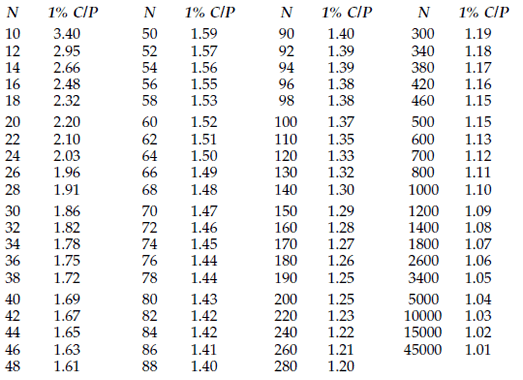Figure 2:  Maximum 1% critical values for the predictability ratio

In the interest of simplicity, figure 2 assumes that either an average range or average standard deviation has been used to compute the limits. (For charts that use a median range, you will need to compute an exact p-value using the stability ratio defined below.)

### Example one

The first example will use the ball-joint socket thickness data. Ninety-six values were collected over the course of one week and organized into 24 subgroups of size 4. The capability and performance indexes were Cp = 1.48, Pp = 1.43, Cpk = 0.95, and Ppk = 0.92. Thus the predictability ratio is: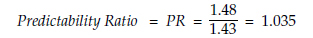For N = 96 values the maximum 1-percent critical value is 1.38. Since the observed predictability ratio of 1.035 is smaller than the 1-percent critical value of 1.38, these data have a p-value larger than 1 percent, and this process might be predictable. The average and range chart in figure 3 confirms that this process was indeed operated predictably during the baseline period.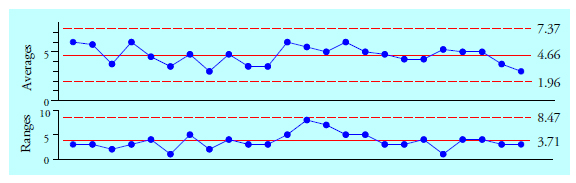Figure 3:  Average and range chart for ball-joint socket thicknesses

### Example two

The creel yield data for one week consist of 33 values placed on an XmR chart. The capability and performance indexes are Cp = 5.38, Pp = 2.40, Cpk = 2.00, and Ppk = 0.90. Thus the predictability ratio is:With N = 33 values we use the maximum critical value for N = 32 values, which is 1.82. Since our predictability ratio of 2.24 exceeds this critical value of 1.82, we know that these data have a p-value that is smaller than 1 percent, and this process is very unlikely to have been operated predictably. The XmR chart in figure 4 confirms this interpretation.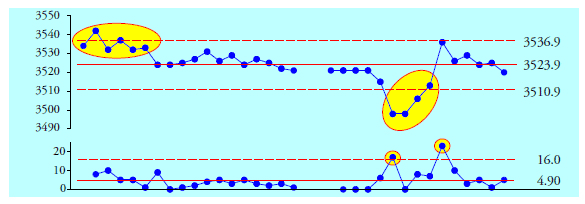Figure 4:  XmR chart for creel yields

Inspection of the formulas given earlier for the capability and performance indexes will quickly reveal that the predictability ratio may be computed using any one of three ratios: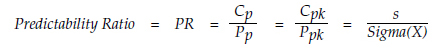When computing a ratio of ratios it is possible for round-off to produce small differences in the results from the different formulas above. When no specifications are given you can still compute a predictability ratio by dividing the global standard deviation statistic, s, by your within-subgroup measure of dispersion, Sigma(X). Common within-subgroup formulas for Sigma(X) are:where d2, d4, and c4 are the usual bias correction factors found in most statistical process control (SPC) books.

### Example three

The camshaft bearing diameter data consist of 50 values placed on an XmR chart. The average moving range is 1.510. Dividing by the bias correction factor of d2 = 1.128 we get a Sigma(X) of 1.3388. The global standard deviation statistic is s = 1.6807. Thus, the predictability ratio is: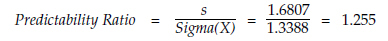From figure 2, the maximum 1-percent critical value for N = 50 is 1.59. Since the predictability ratio of 1.255 is less than this critical value we conclude that the p-value for these data is greater than 0.01. However, this large p-value does not guarantee that this process was operated predictably. It just means that this numerical summary does not provide strong evidence of unpredictability (notice the double negative).

The X chart for the camshaft bearing diameters in figure 5 is much more informative than the predictability ratio. While this process is not terribly unpredictable, it does show evidence of occasional excursions. Since each point on the chart represents 50 parts produced, these excursions represent potential problems.Figure 5:  X  chart for camshaft bearing diameter data

The predictability ratio uses values that are commonly available to provide a quick check on the predictability of your process. Knowledge that the p-value is less than one percent is sufficient to provide reasonable certainty that your process is not being operated up to its full potential. However, if an exact p-value is desired you will need to use the stability ratio.

### The stability ratio

In 2006, Brenda Ramirez and George Runger suggested using the square of the predictability ratio as a measure of process stability over time. They noted that the stability ratio, SR, defined as: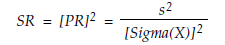will behave as a pseudo-F statistic. The numerator degrees of freedom for this F-distribution will be [N–1]. The denominator degrees of freedom will be the degrees of freedom for the within-subgroup statistic used to compute Sigma(X). So an exact p-value will depend upon three values: the value of the stability ratio, SR; the numerator degrees of freedom, [N–1]; and the denominator degrees of freedom based on how we computed Sigma(X). The next three sections will provide ways to find the denominator degrees of freedom.

### Case one: Sigma(X) is based on the average range

When we use the average range (or the average moving range) to compute Sigma(X) for a baseline period consisting of k subgroups of size n, we can look up the degrees of freedom from figure 6, or approximate them using the formulas in the last row.Figure 6:  Denominator degrees of freedom based on the average range statistic

The formulas in the last row allow you to extend the table in figure 6 to larger numbers of subgroups. For XmR charts the degrees of freedom for the average moving range can be approximated by the formula:### Case two: Sigma(X) is based on the average standard deviation

When we use the average standard deviation statistic to compute Sigma(X) for a baseline period consisting of k subgroups of size n, we can look up the degrees of freedom from figure 7, or approximate them using the formulas in the last row.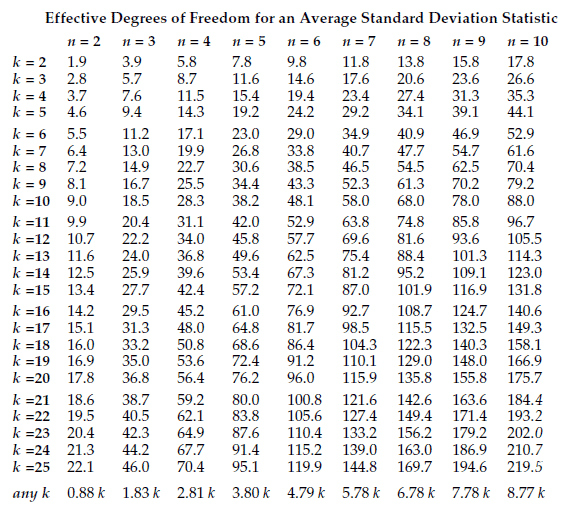Figure 7:  Denominator d.f. based on the average standard deviation statistic

The formulas in the last row allow you to extend the table in figure 7 to larger numbers of subgroups. For subgroup sizes greater than 10 the degrees of freedom for the average standard deviation statistic may be approximated using the formula: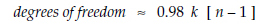### Case three: Sigma(X) is based on the median range

When we use a median range (or a median moving range) to compute Sigma(X) for a baseline period consisting of k subgroups of size n, we can look up the degrees of freedom from figure 8, or approximate them using the formulas in figure 9.Figure 8:  Denominator degrees of freedom based on the median range statistic

The stair-step nature of the values in each column of figure 8 complicates the problem of approximating the degrees of freedom. For XmR charts (where n = 1) the formulas will be given in terms of odd values of k, and the degrees of freedom for an even value of k will be approximately the same as for k–1.

For average and range charts (where n ≥ 2) the formulas will be given in terms of even values for k, and the degrees of freedom for odd values of k will be approximately the same as for k–1.

The formulas for approximating and extending figure 8 may be found in figure 9.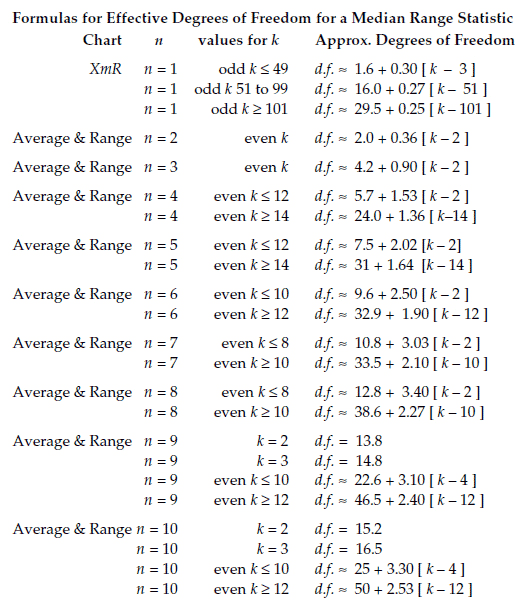Figure 9:  Degrees of freedom formulas for median range statistics

### Finding an exact p-value

Thus, the p-value for your process behavior chart will depend upon three quantities: the observed value for the stability ratio, SR; the numerator degrees of freedom, [N–1]; and the appropriate denominator degrees of freedom from figures 6, 7, 8, or 9.

In Excel you can use the FDIST (F-distribution) function to obtain the p-value for the computed stability ratio by entering the following formula in a cell:and Excel will return the p-value.

### Exact p-value for example one

Recall that the ball-joint socket data were organized in an average and range chart with n = 4, k = 24, and N = 96. The stability ratio is: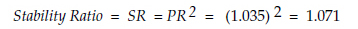The numerator d.f. is N–1 = 95, and from figure 6 the denominator d.f. is 66. From these three quantities we get a p-value of 0.387. This value may be interpreted as the likelihood that these baseline data came from a predictable process. This was confirmed by what we found in figure 3.

### Exact p-value for example two

Recall that the creel yield data were placed on an XmR chart with n = 1, k = 33, and so N = 33. The stability ratio is: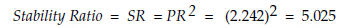The numerator d.f. is N–1 = 32, and from the formula following figure 6, the denominator d.f. is 19.8. From these three quantities we get a p-value of 0.00025 for this chart. This tiny p-value represents the astronomically remote likelihood that these baseline data came from a predictable process. Thus, we conclude that it is more likely that these data came from an unpredictable process, which is what we found in figure 4.

### Exact p-value for example three

The camshaft bearing diameter data were placed on an XmR chart with n = 1, k = 50, and so  N = 50.  The stability ratio is:The numerator d.f. is N–1 = 49, and from the formula following figure 6, the denominator d.f. is 30.0. From these three quantities we get a p-value of 0.093 for this chart. A predictable process with a baseline of 50 values could have a stability ratio this size or greater about 9 percent of the time. So while the stability ratio does not provide strong evidence of unpredictability, the X chart in figure 5 shows 3 out of 50 values outside the limits, and this process is properly judged to be unpredictable. This is why only very small p-values provide an unequivocal interpretation, and larger p-values are ambiguous.

### The origin of figure 2

Instead of computing specific p-values, figure 2 provides cut-offs that allow you to classify a p-value as larger or smaller than 0.01. To get the values in figure 2 the 1-percent critical values for the stability ratio were computed for different combinations of n and k. Next these critical values were converted into critical values for the predictability ratio and plotted vs. the value for N. For each value of N these critical values turned out to all be very similar. This similarity allowed the simplification of tabling the maximum 1-percent critical value for each value of N to produce the table in figure 2.

When the critical values for limits based on a median range were added to the mix the strong similarity between critical values observed earlier for each value of N was no longer present. (This was due to the substantial differences in degrees of freedom when using median ranges.) So, when using a median range you cannot use figure 2 to characterize the predictability ratio, but will instead need to find an exact p-value using the stability ratio.

### Summary

The p-value for either the predictability ratio or the stability ratio may be used as a one-number summary to characterize the predictability of a process during a baseline period. These ratios may be computed from capability and performance ratios, or they may be computed directly using the global standard deviation statistic and a within-subgroup measure of dispersion based on the average range, the median range, or the average standard deviation.

The predictability ratio, PR, may be used with the table in figure 2 to characterize the p-value as being larger or smaller than 0.01. When the p-value can be shown to be smaller than 0.01 the unpredictability of the process is beyond reasonable doubt.

The square of the predictability ratio is known as the stability ratio, SR. It can be used to obtain an exact p-value using an F-distribution. This requires finding the denominator degrees of freedom, but the values and formulas in figures 6, 7, 8, and 9 simplify this computation. Finding an exact p-value for the stability ratio provides a numerical summary that quantifies in a general way the likelihood that a particular process is being operated predictably.

While a very small p-value is a sure sign of an unpredictable process, a larger p-value is no guarantee of predictability. This is because no formula or algorithm can detect all types of unpredictable behavior. Every formula will have its blind spots, and aggregate summaries like the stability ratio are no exception.

When your stability ratio or predictability ratio has a small p-value you should know that the summary and descriptive statistics you have computed using your historical baseline data will not characterize the future operation of the process. When the p-value is small it means that the process average and the process standard deviation are changing over time, and thus the capability and performance indexes will also be changing. However, when your current p-value is small, you can expect that future p-values for your process are likely to remain small until you take action to operate the process predictably.

When process behavior charts are used as a sequential procedure to listen to the voice of the process in real time, reasonable baselines will generally contain somewhere between 20 and 150 data. However, many software packages dump all of the historical data into the baseline. This practice treats a process behavior chart as a one-time analysis procedure. When this happens you may have baselines consisting of thousands of data. This is why figure 2 contains such large values for N

There are two drawbacks to using a process behavior chart as a one-time analysis. First, when your baseline contains more than a few hundred data you are virtually guaranteed to find a small p-value. Second, by the time you have found the signals you will usually have forgotten what happened to create the shifts and spikes seen on your chart, making it impossible for you to use the chart to learn how to improve your process.

However, now you have a p-value for the monthly report that quantifies the probability that your process was operated predictably. The next step is to figure out what to do about your unpredictable processes.

### Discuss### Donald J. Wheeler

Find out about Dr. Wheeler’s virtual seminars for 2022 at www.spcpress.com. Dr. Wheeler is a fellow of both the American Statistical Association and the American Society for Quality who has taught more than 1,000 seminars in 17 countries on six continents. He welcomes your questions; you can contact him at djwheeler@spcpress.com.